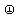# U+29B9 CIRCLED PERPENDICULAR

U+29B9 was added to Unicode in version 3.2 (2002). It belongs to the blockMiscellaneous Mathematical Symbols-B in the Basic Multilingual Plane.

This character is a Math Symbol and is commonly used, that is, in no specific script.

The glyph is not a composition. It has a Neutral East Asian Width. In bidirectional context it acts as Other Neutral and is not mirrored. In text U+29B9 behaves as Alphabetic regarding line breaks. It has type Other for sentence and Other for word breaks. The Grapheme Cluster Break is Any.

In elementary geometry, the property of being perpendicular (perpendicularity) is the relationship between two lines which meet at a right angle (90 degrees). The property extends to other related geometric objects.

A line is said to be perpendicular to another line if the two lines intersect at a right angle. Explicitly, a first line is perpendicular to a second line if (1) the two lines meet; and (2) at the point of intersection the straight angle on one side of the first line is cut by the second line into two congruent angles. Perpendicularity can be shown to be symmetric, meaning if a first line is perpendicular to a second line, then the second line is also perpendicular to the first. For this reason, we may speak of two lines as being perpendicular (to each other) without specifying an order.

Perpendicularity easily extends to segments and rays. For example, a line segment is perpendicular to a line segment if, when each is extended in both directions to form an infinite line, these two resulting lines are perpendicular in the sense above. In symbols, means line segment AB is perpendicular to line segment CD. The point B is called a foot of the perpendicular from A to segment , or simply, a foot of A on .

A line is said to be perpendicular to a plane if it is perpendicular to every line in the plane that it intersects. Note that this definition depends on the definition of perpendicularity between lines.

Two planes in space are said to be perpendicular if the dihedral angle at which they meet is a right angle (90 degrees).

Perpendicularity is one particular instance of the more general mathematical concept of orthogonality; perpendicularity is the orthogonality of classical geometric objects. Thus, in advanced mathematics, the word "perpendicular" is sometimes used to describe much more complicated geometric orthogonality conditions, such as that between a surface and its normal.

## Representations

System Representation
10681
UTF-8 E2 A6 B9
UTF-16 29 B9
UTF-32 00 00 29 B9
URL-Quoted %E2%A6%B9
HTML-Escape &#x29B9;
Wrong windows-1252 Mojibake â¦¹
HTML-Escape &operp;

## Complete Record

Property Value
Age (age) 3.2
Unicode Name (na) CIRCLED PERPENDICULAR
Unicode 1 Name (na1)
Block (blk) Misc_Math_Symbols_B
General Category (gc) Math Symbol
Script (sc) Common
Bidirectional Category (bc) Other Neutral
Combining Class (ccc) Not Reordered
Decomposition Type (dt) None
Decomposition Mapping (dm)Lowercase (Lower)
Simple Lowercase Mapping (slc)Lowercase Mapping (lc)Uppercase (Upper)
Simple Uppercase Mapping (suc)Uppercase Mapping (uc)Simple Titlecase Mapping (stc)Titlecase Mapping (tc)Case Folding (cf)ASCII Hex Digit (AHex)
Alphabetic (Alpha)
Bidi Control (Bidi_C)
Bidi Mirrored (Bidi_M)
Bidi Paired Bracket (bpb)Bidi Paired Bracket Type (bpt) None
Cased (Cased)
Composition Exclusion (CE)
Case Ignorable (CI)
Full Composition Exclusion (Comp_Ex)
Changes When Casefolded (CWCF)
Changes When Casemapped (CWCM)
Changes When NFKC Casefolded (CWKCF)
Changes When Lowercased (CWL)
Changes When Titlecased (CWT)
Changes When Uppercased (CWU)
Dash (Dash)
Deprecated (Dep)
Default Ignorable Code Point (DI)
Diacritic (Dia)
East Asian Width (ea) Neutral
Extender (Ext)
FC NFKC Closure (FC_NFKC)Grapheme Cluster Break (GCB) Any
Grapheme Base (Gr_Base)
Grapheme Extend (Gr_Ext)
Hex Digit (Hex)
Hangul Syllable Type (hst) Not Applicable
Hyphen (Hyphen)
ID Continue (IDC)
Ideographic (Ideo)
ID Start (IDS)
IDS Binary Operator (IDSB)
IDS Trinary Operator and (IDST)
InMC (InMC)
Indic Positional Category (InPC) NA
Indic Syllabic Category (InSC) Other
ISO 10646 Comment (isc)
Joining Group (jg) No_Joining_Group
Join Control (Join_C)
Jamo Short Name (JSN)
Joining Type (jt) Non Joining
Line Break (lb) Alphabetic
Logical Order Exception (LOE)
Math (Math)
Noncharacter Code Point (NChar)
NFC Quick Check (NFC_QC) Yes
NFD Quick Check (NFD_QC) Yes
NFKC Casefold (NFKC_CF)NFKC Quick Check (NFKC_QC) Yes
NFKD Quick Check (NFKD_QC) Yes
Numeric Type (nt) None
Numeric Value (nv) NaN
Other Alphabetic (OAlpha)
Other Default Ignorable Code Point (ODI)
Other Grapheme Extend (OGr_Ext)
Other ID Continue (OIDC)
Other ID Start (OIDS)
Other Lowercase (OLower)
Other Math (OMath)
Other Uppercase (OUpper)
Pattern Syntax (Pat_Syn)
Pattern White Space (Pat_WS)
Quotation Mark (QMark)
Sentence Break (SB) Other
Simple Case Folding (scf)Script Extension (scx) Common
Soft Dotted (SD)
STerm (STerm)
Terminal Punctuation (Term)
Unified Ideograph (UIdeo)
Variation Selector (VS)
Word Break (WB) Other
White Space (WSpace)
XID Continue (XIDC)
XID Start (XIDS)
Expands On NFC (XO_NFC)
Expands On NFD (XO_NFD)
Expands On NFKC (XO_NFKC)
Expands On NFKD (XO_NFKD)